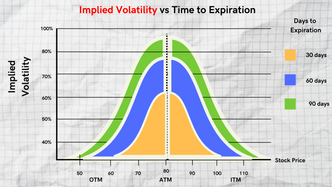top of page
Search

# Understanding Implied Volatility and Its Importance in Option Trading

Hey, hey, hey! Implied volatility, now that's something we need to talk about! It's a key factor in the price of options and can help traders make informed investment decisions. In this article, we break down everything you need to know about implied volatility - how it's calculated, its significance in option trading, and how it impacts pricing. So if you're looking to level up your options trading game, you definitely don't want to miss out on this. Read on to get all the deets!(Source:TheOptionPlaybook)

## What is Implied Volatility?

Implied volatility is the expected volatility of an underlying asset derived from the option's market price. It is a measure of uncertainty in the future price of the underlying asset, and the higher the implied volatility, the greater the expected price movement of the underlying asset.

### How is Implied Volatility Calculated?

Implied volatility is calculated by inputting market data, including the option's current price, the strike price, time to expiration, interest rates, and the current price of the underlying asset, into an options pricing model. The most commonly used pricing model is the Black-Scholes model. Once the market data is inputted, the pricing model calculates the implied volatility of the option.

### The Importance of Implied Volatility in Option Trading

Implied volatility is a critical factor in determining the price of an option. Options with high implied volatility have a higher premium as there is a greater expectation of price movement in the underlying asset. Conversely, options with low implied volatility have a lower premium, as there is a lower expectation of price movement.(Source:InvestingFuse)

Implied volatility also plays a crucial role in option strategies. Traders often use implied volatility to determine the best options trading strategies for the current market conditions. For example, if the implied volatility is high, traders may consider selling options, while if it is low, they may consider buying options.

In addition, implied volatility can help traders predict the potential price movement of an underlying asset. If the implied volatility is high, it suggests that the market expects a significant price movement in the underlying asset, which traders may use to their advantage.

### Conclusion

Implied volatility represents the market's expectation of future volatility, which is a critical factor in determining the price of an option. Traders use implied volatility to determine the best options trading strategies and predict potential price movements of an underlying asset. By understanding the concept of implied volatility and its significance in option trading, traders can make more informed investment decisions.